# LOOKUP Function

The LOOKUP function returns the value in a row (or column) after finding a matching value in a column (or row).
XLOOKUP was added in Excel 2021 to replace this function.
This function is not case sensitive.
In fact before we try and understand this function we need to make a small modification to the tooltip.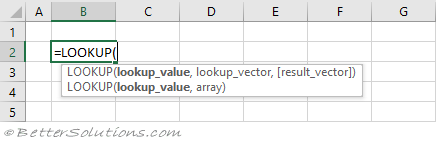The Vector syntax for this function is the one used in 99% of cases, so lets assume that this is the only one that exists.
The other one was originally provided for backwards compatibility and is rarely seen or used.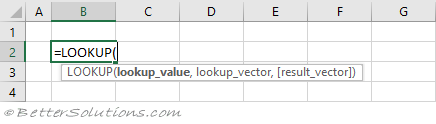### Return the Exact Match (unique values)

The table has to be sorted when you are looking for a unique exact match.
If there is more than one occurrence, the last occurrence is used.
The table has been sorted by Country and Sales into ascending order.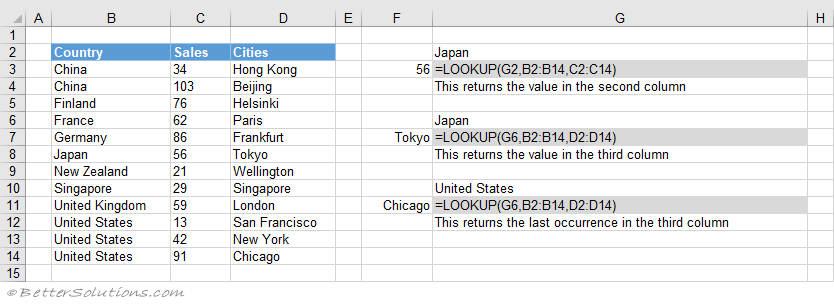### Return the Exact Match (item does not exist)

The table has to be sorted when you are looking for a unique exact match.
When a value is not in the list, you might get #N/A or something unexpected.
When the item does not exist and the "lookup_value" is less than the smallest value in "lookup_vector" #N/A is returned.
The table has been sorted by Country into ascending order.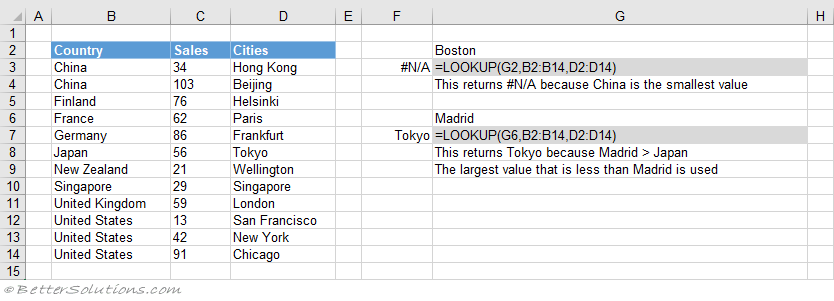### Return the Exact Match (different size vectors)

When the "lookup_vector" and the "result_vector" are different sizes, you might get #N/A or something unexpected.
These three formulas have a "lookup_vector" with length 13 and a "result_vector" with length 2.
When using this function always make sure the vectors are exactly the same size.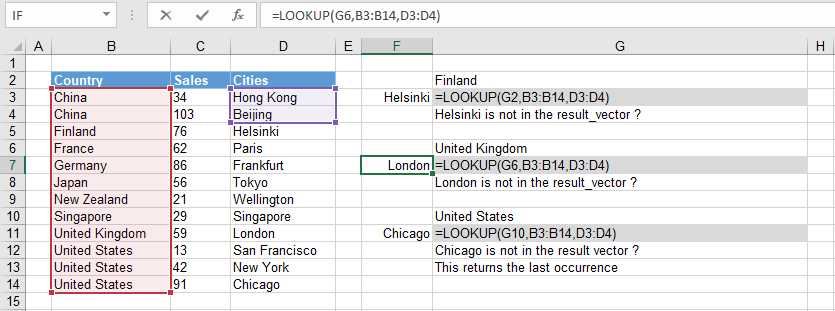### Return the Exact Match (case sensitive)

The LOOKUP function is not case sensitive.
When using this function the table has to be sorted.
The table has been sorted by Country into ascending order.
The "0" passed to the MATCH function means that it will find an exact match.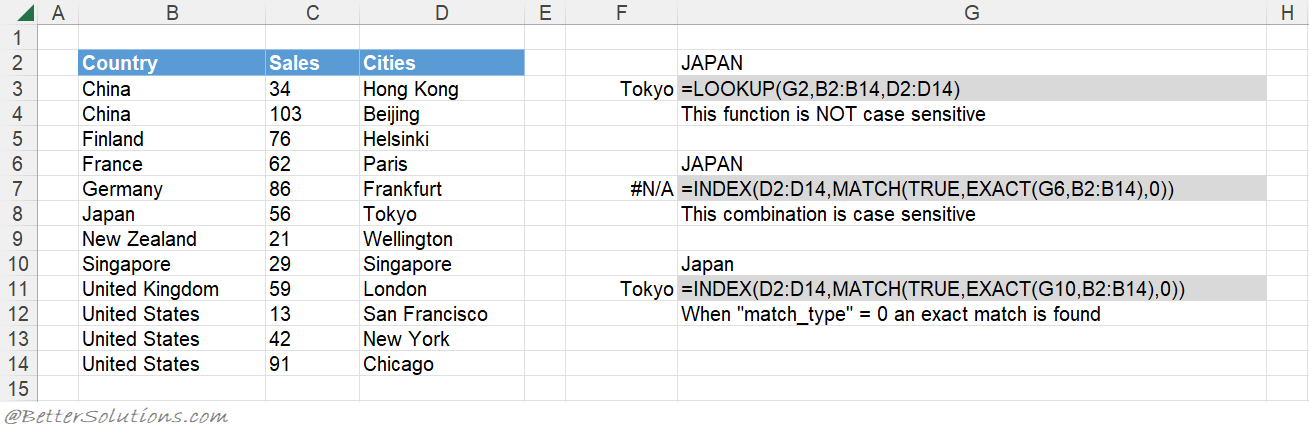### Return the Exact Match (first occurrence, sorted or not sorted)

When using this function the table has to be sorted.
The table has been sorted by Country into ascending order.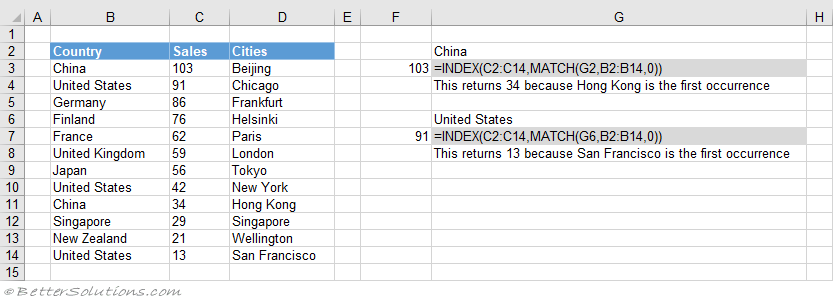### Return the Exact Match (last occurrence, sorted)

When using this function the table has to be sorted.
The table has been sorted by Country and Sales into ascending order.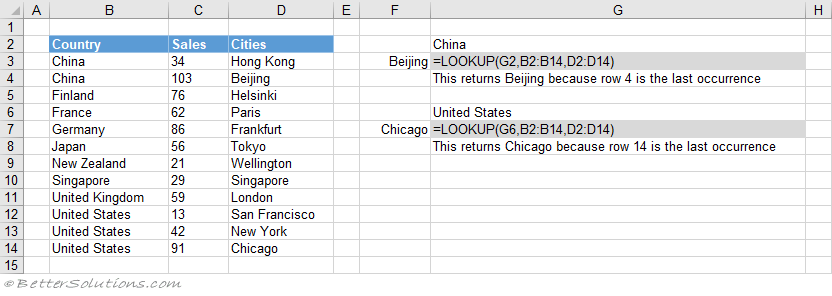### Return the Exact Match (last occurrence, not sorted)

The LOOKUP function uses an array of cell values and its closest match logic to find the last match.
The array eventually contains either 1's or error values and because the "lookup_value" is 2 it will return the position of the last 1 in the array.
You could also use the MAX and SUMPRODUCT functions as an alternative to using the LOOKUP function.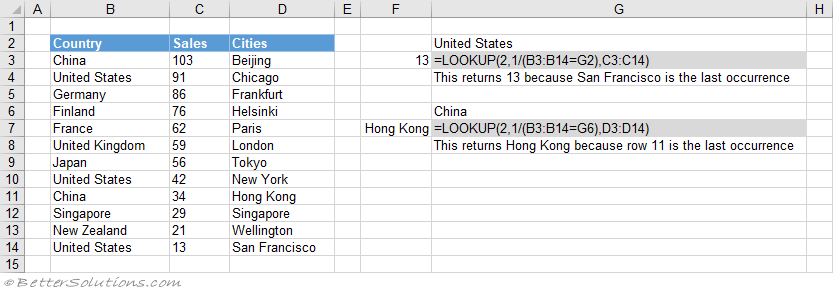### Return the Exact Match (with wildcard *)

An asterisk (*) matches any sequence of characters.

### Return the Exact Match (with wildcard ?)

A question mark (?) matches any single character.

### Return the Exact Match (multiple criteria)

When you want to return a value in the same row after finding matching values in 2 (or more) columns.

### Return the Next Smallest

When using this function the table has to be sorted.
The table has been sorted by Sales into ascending order.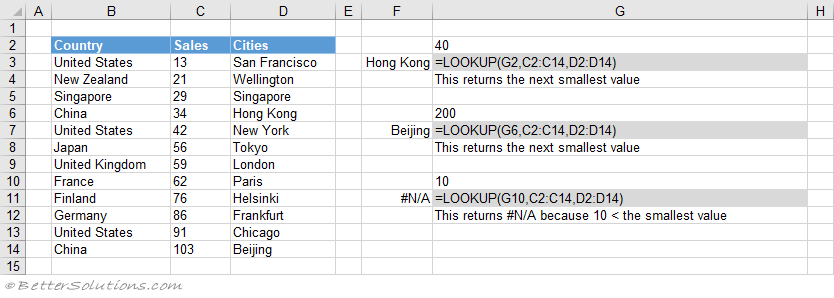### Return the Next Largest

When using this function the table has to be sorted.
The table has been sorted by Sales into descending order.
The "-1" passed to the MATCH function means that it will find the smallest value that is greater than the lookup value.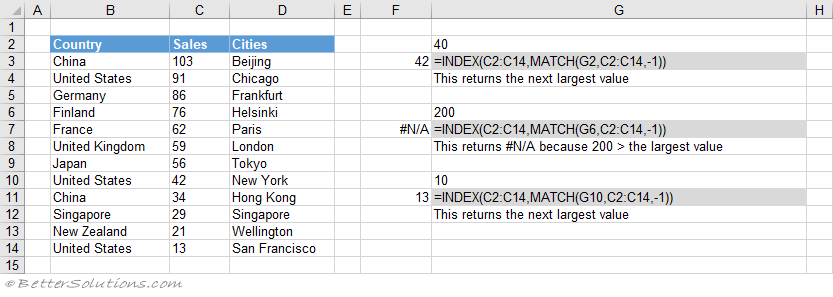### Array Form

To use this syntax your data must be sorted.